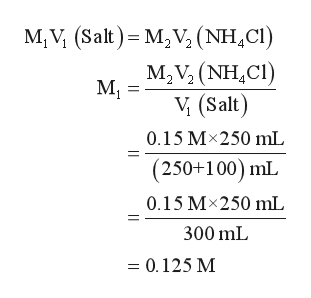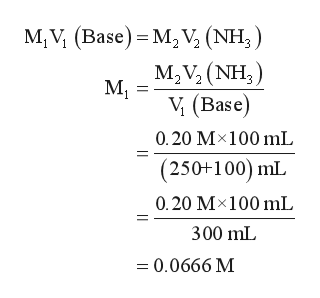# Calculate the pH of a solution formed by mixing 250.0 mL of 0.15 M NH4Cl with 100.0 mL of 0.20 M NH3. The Kb for NH3 is 1.8 x 10-5.

Question
17 views

Calculate the pH of a solution formed by mixing 250.0 mL of 0.15 M NH4Cl with 100.0 mL of 0.20 M NH3. The Kb for NH3 is 1.8 x 10-5.

check_circle

Step 1

Given information:

Volume of NH4Cl solution (V1) =250.0 mL

Volume of NH3 solution (V2) =100.0 mL

Concentration of NH4Cl solution (M1) = 0.15 M

Concentration of NH3 solution (M2) = 0.20 M

Step 2

First the concentration of new solution formed  is calculated as follows:help_outlineImage TranscriptioncloseM, V (Salt) M,V (NH,C) M2V3 (NH,CI) М, V (Salt) 0.15 Mx250 mL (250+100) mL 0.15 Mx250 mL 300 mL = 0.125 M fullscreen
Step 3

Now, the concentration of base is calc...help_outlineImage TranscriptioncloseM, V (Base) M2 V, (NH,) M2V2 (NH3) М, V (Base) 0.20 M100 mL (250+100) mL 0.20 M 100 mL 300 mL =0.0666 M fullscreen

### Want to see the full answer?

See Solution

#### Want to see this answer and more?

Solutions are written by subject experts who are available 24/7. Questions are typically answered within 1 hour.*

See Solution
*Response times may vary by subject and question.
Tagged in

### Chemistry